Next: Tree-level Couplings Up: SUPERSTRING PHENOMENOLOGY AND THE Previous: Other Constructions

# Effective Actions in 4D

We have seen that consistent 4D chiral string models lead to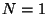supersymmetry. For phenomenological purposes, we are interested in finding the effective action for the light degrees of freedom, that means we want to integrate out all the heavy degrees of freedom at the Planck scale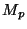and compute the effective couplings among the light states (massless at the Planck scale). This will be a standard field theoretical action withsupersymmetry. The on-shell massless spectrum of these models have the graviton-gravitino multiplet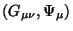, the gauge-gaugino multiplets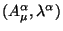and the matter and moduli fields which fit intochiral multiplets of the form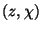except for the dilaton field which together with the antisymmetric tensor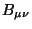belong to a linearmultiplet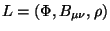. The most general couplings of supergravity to one linear multiplet and several gauge and chiral multiplets is not yet known, although some progress towards its construction has been done recently [30,31]. Nevertheless, as we mentioned in the previous chapter, this field can be dualized to construct the chiral multiplet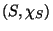with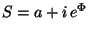and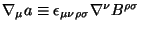. After performing this duality transformation we are lead with asupergravity theory coupled only to gauge and chiral multiplets. The most general such action was constructed more than a decade ago , and therefore it is more convenient in this sense to work with the dual dilaton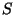rather than the stringy one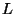. Although, the partial knowledge about the lagrangian in terms ofis enough to understand most of the results we will mention next , we will only mention the approach with the fieldwhich is the most commonly used. The general Lagrangian couplingsupergravity to gauge and chiral multiplets depends on three arbitrary functions of the chiral multiplets:

(1)The Kähler potential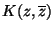which is a real function. It determines the kinetic terms of the chiral fields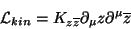(14)

with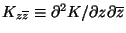.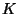is called Kähler potential because the manifold of the scalar fields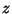is Kähler, with metric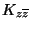.

(2)The superpotential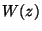which is a holomorphic function of the chiral multiplets (it does not depend on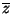).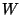determines the Yukawa couplings as well as the-term part of the scalar potential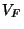(known as-term because it originates after eliminating auxiliary fields associated to the chiral multiplets which are usually called):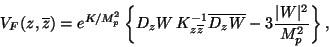(15)

with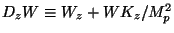. Here and in what follows, the internal indices labelling different chiral multiplets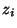are not explicitly written.

(3)The gauge kinetic function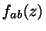which is also holomorphic. It determines the gauge kinetic terms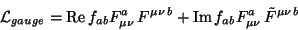(16)

it also contributes to gaugino masses and the gauge part of the scalar potential (coming from eliminating the auxiliary fields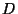of the gauge multiplets and referred to as-terms).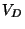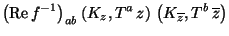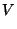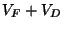(17)

The problem posed in this section is: given a 4D string model, calculate the functions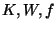. In order to do that let us separate the fields into the moduli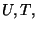the dilaton,and the matter fields charged under the gauge group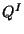. Most of the structure of the couplings depends on the model. But there are some couplings which are model independent. To extract them, the best procedure is to use all the symmetries at hand. For this let us remark that 4D strings are controlled by two perturbation expansions. One is the expansion in the sigma-model (2D worldsheet) which is governed by the expectation value of a modulus field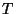(the size of the extra dimensions). Whereas proper string perturbation theory is governed by the dilaton field.

SubsectionsNext: Tree-level Couplings Up: SUPERSTRING PHENOMENOLOGY AND THE Previous: Other Constructions
root 2001-01-22